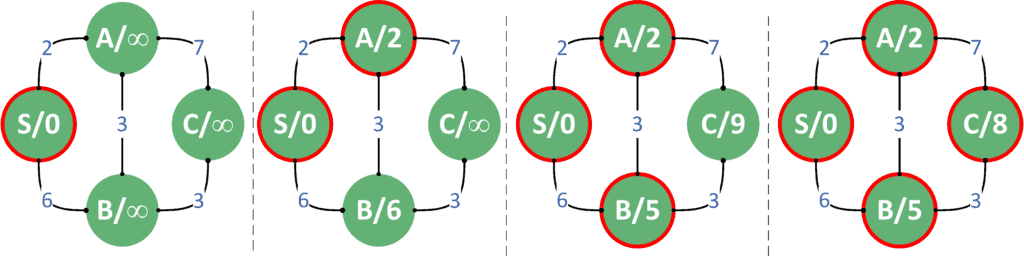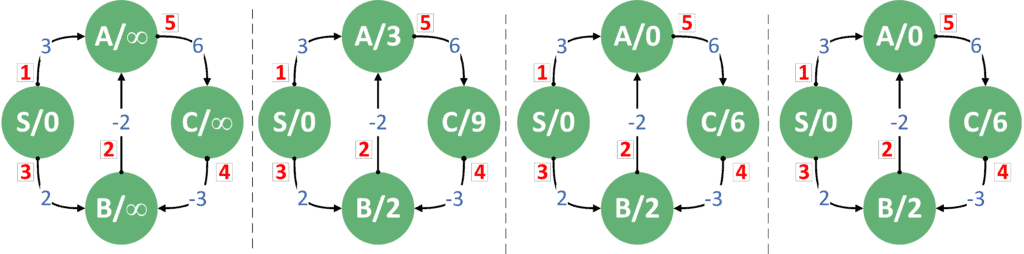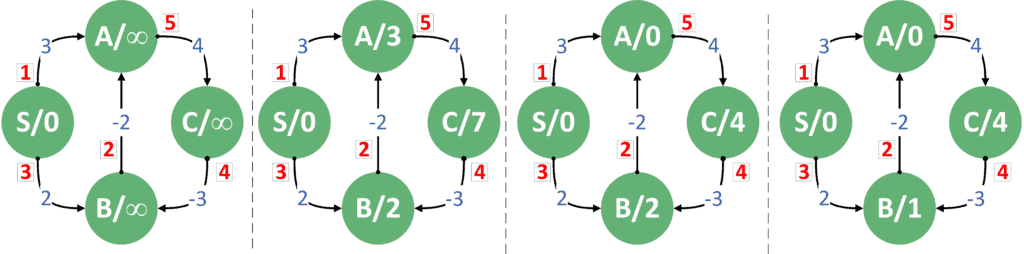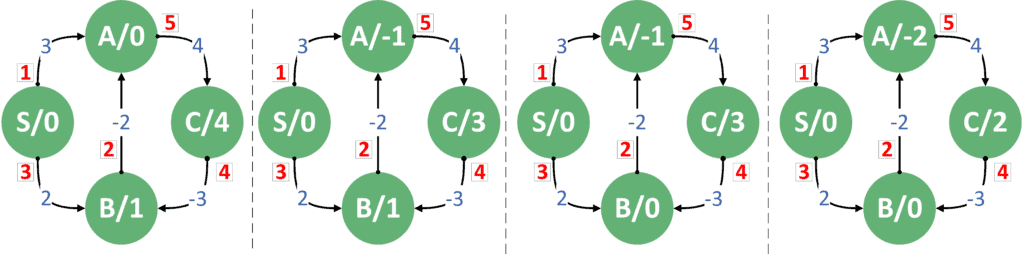## 1. Overview

In this tutorial, we’ll give an overview of the Dijkstra and Bellman-Ford algorithms. We’ll discuss their similarities and differences.

Then, we’ll summarize when to use each algorithm.

## 2. Dijkstra’s Algorithm

Dijkstra’s algorithm is one of the SSSP (Single Source Shortest Path) algorithms. Therefore, it calculates the shortest path from a source node to all the nodes inside the graph.

Although it’s known that Dijkstra’s algorithm works with weighted graphs, it works with non-negative weights for the edges. We’ll explain the reason for this shortly.

### 2.1. Theoretical Idea

In Dijkstra’s algorithm, we start from a source node and initialize its distance by zero. Next, we push the source node to a priority queue with a cost equal to zero.

After that, we perform multiple steps. In each step, we extract the node with the lowest cost, update its neighbors’ distances, and push them to the priority queue if needed. Of course, each of the neighboring nodes is inserted with its respective new cost, which is equal to the cost of the extracted node plus the edge we just passed through.

We continue to visit all nodes until there are no more nodes to extract from the priority queue. Then, we return the calculated distances.

The complexity of Dijkstra’s algorithm is, whereis the number of nodes, andis the number of edges in the graph.

### 2.2. Proof of Concept

In Dijkstra’s algorithm, we always extract the node with the lowest cost. We can prove the correctness of this approach in the case of non-negative edges.

Suppose the node with the minimum cost is. Also, suppose we want to extract some other nodethat has a higher cost than. In other words, we have:We can’t possibly reachwith a lower cost if we extractedfirst. The reason behind this is thatitself has a higher cost. Therefore, any path that takes us tostarting fromwill have a cost equal to the cost ofplus the distance fromto. In other words, we are trying to prove that:However, we already know thatis smaller than. Sincehas a non-negative weight, the last equation can never come true. Therefore, it’s always optimal to extract the node with the minimum cost.

### 2.3. Limitations

So, we proved the optimality of Dijkstra’s algorithm. However, to do this, we assumed that all the edges have non-negative weights. Therefore,will always be non-negative as well.

When working with graphs that have negative weights, Dijkstra’s algorithm fails to calculate the shortest paths correctly. The reason is thatmight be negative, which will make it possible to reachfromat a lower cost. Therefore, we can’t prove the optimality of choosing the node that has the lowest cost.

### 2.4. Advantages and Disadvantages

The main advantage of Dijkstra’s algorithm is its considerably low complexity, which is almost linear. However, when working with negative weights, Dijkstra’s algorithm can’t be used.

Also, when working with dense graphs, whereis close to, if we need to calculate the shortest path between any pair of nodes, using Dijkstra’s algorithm is not a good option.

The reason for this is that Dijkstra’s time complexity is. Sinceequals almost, the complexity becomes.

When we need to calculate the shortest path between every pair of nodes, we’ll need to call Dijkstra’s algorithm, starting from each node inside the graph. Therefore, the total complexity will become.

The reason why this is not a good enough complexity is that the same can be calculated using the Floyd-Warshall algorithm, which has a time complexity of. Hence, it can give the same result with lower complexity.

## 3. Bellman-Ford Algorithm

As with Dijkstra’s algorithm, the Bellman-Ford algorithm is one of the SSSP algorithms. Therefore, it calculates the shortest path from a starting source node to all the nodes inside a weighted graph. However, the concept behind the Bellman-Ford algorithm is different from Dijkstra’s.

### 3.1. Theoretical Idea

In the Bellman-Ford algorithm, we begin by initializing all the distances of all nodes with, except for the source node, which is initialized with zero. Next, we performsteps.

In each step, we iterate over all the edges inside the graph. For each edge from nodeto, we update the respective distances ofif needed. The new possible distance equals to the distance ofplus the weight of the edge betweenand.

Aftersteps, all the nodes will have the correct distance, and we stop the algorithm.

The Bellman-Ford algorithm’s time complexity is, whereis the number of vertices, andis the number of edges inside the graph. The reason for this complexity is that we performsteps. In each step, we visit all the edges inside the graph.

### 3.2. Proof of Concept

The Bellman-Ford algorithm assumes that aftersteps, all the nodes will surely have correct distances. Let’s prove this assumption.

Any acyclic path inside the graph can have at mostnodes, which means it hasedges. If a path has more thanedges, it means that the path has a cycle because it has more thannodes. Therefore, it must visit the same node more than once.

We can guarantee that any shortest path won’t go through cycles. Otherwise, we could have removed the cycle, and gained a better path.

Going back to the Bellman-Ford algorithm, we can guarantee that aftersteps, the algorithm will cover all the possible shortest paths. Therefore, the algorithm is guaranteed to give an optimal solution.

### 3.3. Limitations

So, we proved that the Bellman-Ford algorithm gives an optimal solution for the SSSP problem. However, the first limitation to our proof is that going through a cycle could improve the shortest path!

The only case this is correct is when we have a cycle that has a negative total sum of edges. In that case, we usually can’t calculate the shortest path because we can always get a shorter path by iterating one more time inside the cycle. Therefore, the term shortest path loses its meaning.

However, even if the graph has negative weights, our proof holds still as long as we don’t have negative cycles.

In fact, we can use the Bellman-Ford algorithm to check for the existence of negative cycles. The only update we need to do is to save the distances we calculated after performingsteps. Next, we perform one more step (step number) the same way we did before.

After that, we check whether we have a node that got a better path. If so, then we must have at least one negative cycle that is causing this node to get a shorter path.

The second limitation is related to undirected graphs. Although it’s true that we can always transform an undirected graph to a directed graph, Bellman-Ford fails to handle undirected graphs when it comes to negative weights.

As far as the Bellman-Ford algorithm is concerned, if the edge betweenandhas a negative weight, we now have a negative cycle. The cycle is formed by going fromtoand back to, which has a weight equal to twice the edge betweenand.

### 3.4. Advantages and Disadvantages

The main advantage of the Bellman-Ford algorithm is its capability to handle negative weights. However, the Bellman-Ford algorithm has a considerably larger complexity than Dijkstra’s algorithm. Therefore, Dijkstra’s algorithm has more applications, because graphs with negative weights are usually considered a rare case.

As mentioned earlier, the Bellman-Ford algorithm can handle directed and undirected graphs with non-negative weights. However, it can only handle directed graphs with negative weights, as long as we don’t have negative cycles.

Also, we can use the Bellman-Ford algorithm to check the existence of negative cycles, as already mentioned.

## 4. Non-Negative Weights Example

Let’s take an example of a graph that has non-negative weights and see how Dijkstra’s algorithm calculates the shortest paths.First, we pushto a priority queue and set its distance to zero. Next, we extract it, visit its neighbors, and update their distances. After that, we extractfrom the priority queue since it has the shortest distance, update its neighbors, and push them to the priority queue.

The next node to be extracted issince it has the shortest path. As before, we update its neighbors and push them to the queue if needed. Finally, we extractfrom the queue, which now has its correct shortest path.

It’s worth noting that bothandhad their distances updated more than once. In the case of, we first set its distance equal to 6. However, when we extracted, we updated the distance ofwith the better path of distance 5.

The same holds for. When we extracted, we updated its distance to be equal to 9. However, when we extracted, we found a better path to, which has a distance equal to 8.

In each step, the only distance we were certain about is the lowest one. Therefore, we kept extracting it from the priority queue and updating its neighbors. When we didn’t have more nodes to extract from the priority queue, all the shortest paths had already been calculated correctly.

## 5. Negative Weights Example

Now, let’s have a look at an example of a graph containing negative weights, but without negative cycles.The red number near each edge shows its respective order. We performed three steps. In each step, we iterated over the edges by their order and updated the distances.

First, we updated the distance offrom the first edge, updated the distance offrom the third edge, and updated the distance offrom the fifth edge. Next, we updated the distance offrom the second edge and updated the distance offrom the fifth edge. On the third step, we didn’t update any distances.

We can notice that performing any number of steps after thesteps we already performed won’t change any distance. Therefore, we guarantee that the graph doesn’t contain negative cycles.

## 6. Negative Cycles Example

Now let’s look at an example that has negative cycles and explain how the Bellman-Ford algorithm detects negative cycles.In the first step, we updated the distance offrom the first edge, the distance offrom the third edge, and the distance offrom the fifth edge. Next, we updated the distance offrom the second edge and the weight offrom the fifth edge. Third, we updated the weight offrom the fourth edge.

However, unlike the previous example, this example contains a negative cycle. The negative cycle isbecause the sum of weights on this cycle is -1.

Let’s perform a few more iterations and see if the Bellman-Ford algorithm can detect it.The first graph contains the resulting distances after performing thesteps. If we performed one more step, we can notice that we update the distance offrom the second edge and the distance offrom the fourth edge. If we kept performing iterations, we’d notice that nodes,, andkept having lower distances because they are inside the negative cycle.

Therefore, since we have at least one node whose distance was updated, we can declare that the graph has negative cycles.

## 7. Comparison

Take a look at the similarities and differences between Dijkstra’s and Bellman-Ford algorithms:As we can see, Dijkstra’s algorithm is better when it comes to reducing the time complexity. However, when we have negative weights, we have to go with the Bellman-Ford algorithm. Also, if we want to know whether the graph contains negative cycles or not, the Bellman-Ford algorithm can help us with that.

Just one thing to remember, in case of negative weights or even negative cycles, the Bellman-Ford algorithm can only help us with directed graphs.

## 8. Conclusion

In this tutorial, we provided an overview of Dijkstra’s and Bellman-Ford algorithms. We listed all the limitations, advantages, and disadvantages of each algorithm. Finally, we compared their strengths and weaknesses.

2 Comments
Inline Feedbacks
View all commentsMarti
8 months ago

I feel that maybe the time complexity of Dijkstra Algorithm is O(E + Vlog(V))Loredana Crusoveanu
7 months ago
Reply to  Marti

Hi Marti,
That’s true when using a Fibonacci heap min-priority queue. However, the originally proposed algorithm used a simple min-priority queue with a time complexity of O(V + E.log(V)) as mentioned in the article.
Cheers!

Comments are closed on this article!